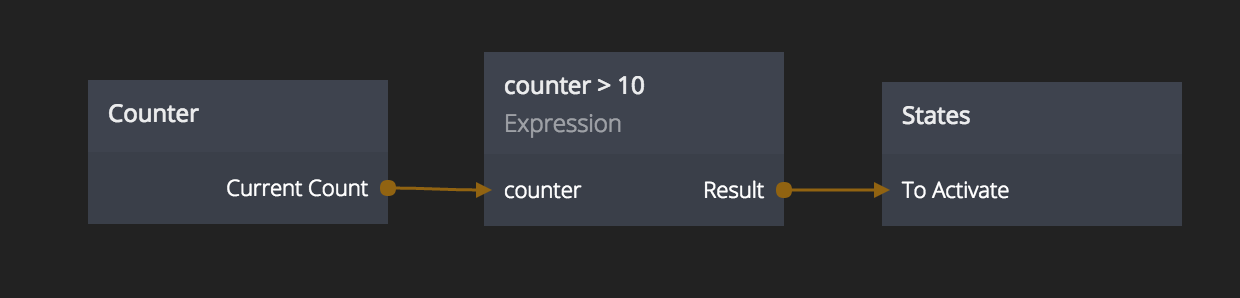# Expression

This node enables you to do your own calculations using simple expressons. Inputs are automatically derived from the expression. An expression set to `a + someName + 1` will get two inputs: one for a and one for someName.The Expression node runs JavaScript under the hood and supports a range of mathematical expressions.

## Built in math functions​

Expressions support the following funcitons:

• `min(a,b)` - Minimum of two values
• `max(a,b)` - Maximum of two values
• `cos(angle)` - The cosine of an angle in radians
• `sin(angle)` - The sine of an angle in radians
• `tan(angle)` - The tangent of an angle in radians
• `sqrt(a)` - Square root of a number
• `pi` - PI in radians
• `round(a)` - Rounds a number to the closest integer
• `floor(a)` - Removes the fractional part of a number
• `ceil(a)` - Rounds a value up to the closest higher integer. E.g. `round(1.1)` equals `2`.
• `abs(a)` - The absolute value of a number. Negative values will become positive.
• `random()` - A random number from 0 to 1

The Expression node is running JavaScript under the hood so in addition to the above functions any standard JavaScript functions will also be valid. The Expression node will add a `return` statement before the expression is executed. `1+2` will be evaluated as `return (1+2)`

## Inputs​

InputsInputs are automatically derived from the expression. An expression set to `a + someName + 1` will get two inputs, one for `a` and one for `someName`.